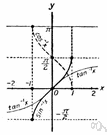# inverse function

(redirected from Inverse (functions))
Also found in: Thesaurus, Encyclopedia.
Related to Inverse (functions): Inverse trigonometric functions

## inverse function

n. Mathematics
A function whose relation to a given function is such that their composite is the identity function. It is often found by interchanging dependent and independent variables.
 Noun 1inverse function - a function obtained by expressing the dependent variable of one function as the independent variable of another; f and g are inverse functions if f(x)=y and g(y)=xfunction, mapping, mathematical function, single-valued function, map - (mathematics) a mathematical relation such that each element of a given set (the domain of the function) is associated with an element of another set (the range of the function)